# Quadratic Functions

Quadratic Functions

In this mini-lesson, we will explore the world of quadratic functions in math. You will get to learn about the graphs of quadratic functions, quadratic functions formula, and other interesting facts around the topic. You can also check out the playful calculators to know more about the lesson and try your hand at solving a few interesting practice questions at the end of the page.

The word "Quadratic" is derived from the word "Quad" which means square.

In other words, a quadratic function is a “ polynomial function of degree 2.”

There are many scenarios where quadratic functions are used.

Did you know that when a rocket is launched, its path is described by the solution of a quadratic function?

##Let's go ahead and learn more about this topic now.

## Lesson Plan

 1 What Is a Quadratic Function? 2 Important Notes on Quadratic Function 3 Solved Examples on Quadratic Function 4 Thinking Out of the Box! 5 Interactive Questions on Quadratic Function

## What Is a Quadratic Function?

### Quadratic Function: Definition

A function of the form $$f(x)=ax^2+bx+c$$, where $$a \neq 0$$ is called a quadratic function.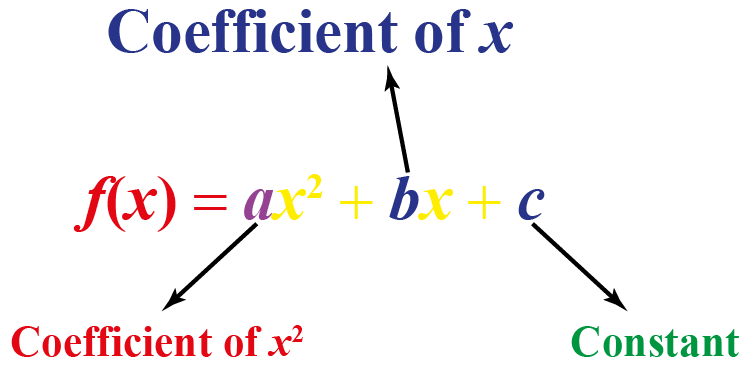Here are some quadratic functions examples.

Functions Values of $$a$$, $$b$$ and $$c$$
1. $$f(x)=8x^2+7x-1$$ \begin{align}a&=8 \\b&=7 \\c&=-1 \end{align}
2. $$f(x)=4x^2-9$$ \begin{align}a&=4 \\b&=0 \\c&=-9 \end{align}
3. $$f(x)=x^2+x$$ \begin{align}a&=1 \\b&=1 \\c&=0 \end{align}
4. $$f(x)=6x-8$$ \begin{align}a&=0 \\b&=6 \\c&=-8 \end{align}

Look at Example 4

Here, $$a = 0$$. Therefore, it is not a quadratic function.

The remaining functions are quadratic function examples.

## What Is an Example of a Quadratic Function?

Sometimes, a quadratic function is not written in its standard form, $$f(x)=ax^2+bx+c$$, and we may have to change it into the standard form.

Here are some examples of functions and their standard forms.

 Functions Steps to follow Standard Form $$f(x)$$=$$(x-1)(x+2)$$ Multiply the factors $$(x-1)$$ and $$(x+2)$$ $$f(x)$$=$$x^2+x-2$$ $$f(x)-x^2$$=$$-3x+1$$ Move the term $$-x^2$$ to the right side of the $$=$$ sign $$f(x)$$=$$x^2-3x+1$$ $$f(x)+5x(x+3)$$=$$12x$$ Expand the bracket on the left side and move $$12x$$ to the left side of the $$=$$ sign $$f(x)$$=$$-5x^2-3x$$ $$f(x)+x^3$$=$$x(x^2+x-3)$$ Expand the bracket on the right side and move $$x^3$$ to right side of the $$=$$ sign $$f(x)$$=$$x^2-3x$$

## Graphs of Quadratic Functions

This is how the quadratic function is represented on a graph.

This curve is called a parabola.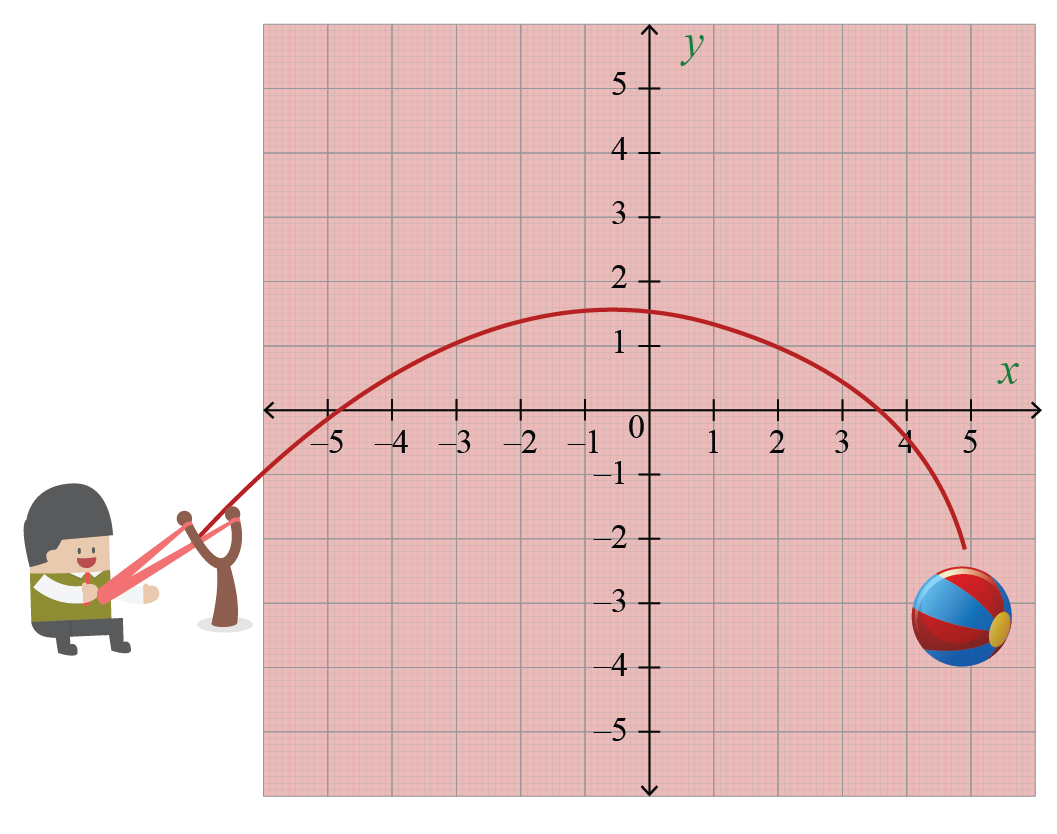### Quadratic Functions: Calculator

Now let’s explore some quadratic equations on a graph using the quadratic function calculators.

Drag the values of $$a$$ to check for the variations of the upward parabola and downward parabola.

Dragging the values of $$b$$ will move the curve either right or left.

Dragging the values of $$c$$ will move the curve either up or down.

## Quadratic Functions: Standard Form

The standard form of a quadratic function is $$f(x)=a(x-h)^{2}+k$$, where $$(h,k)$$ is the vertex of the parabola.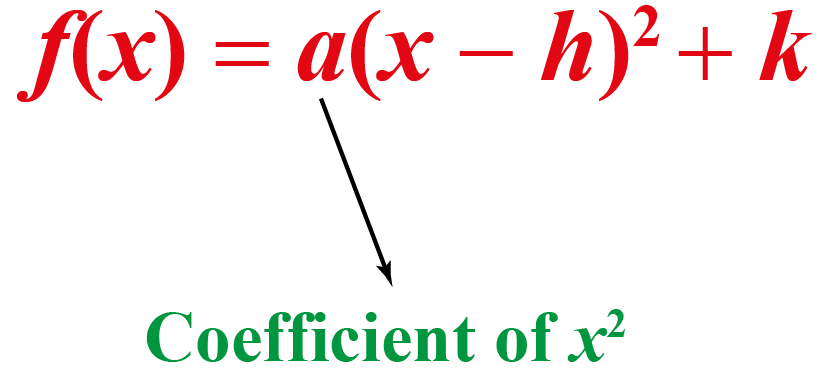This shows the quadratic functions standard form.

If $$a>0$$, then the parabola opens upward.

If $$a<0$$, then the parabola opens downward.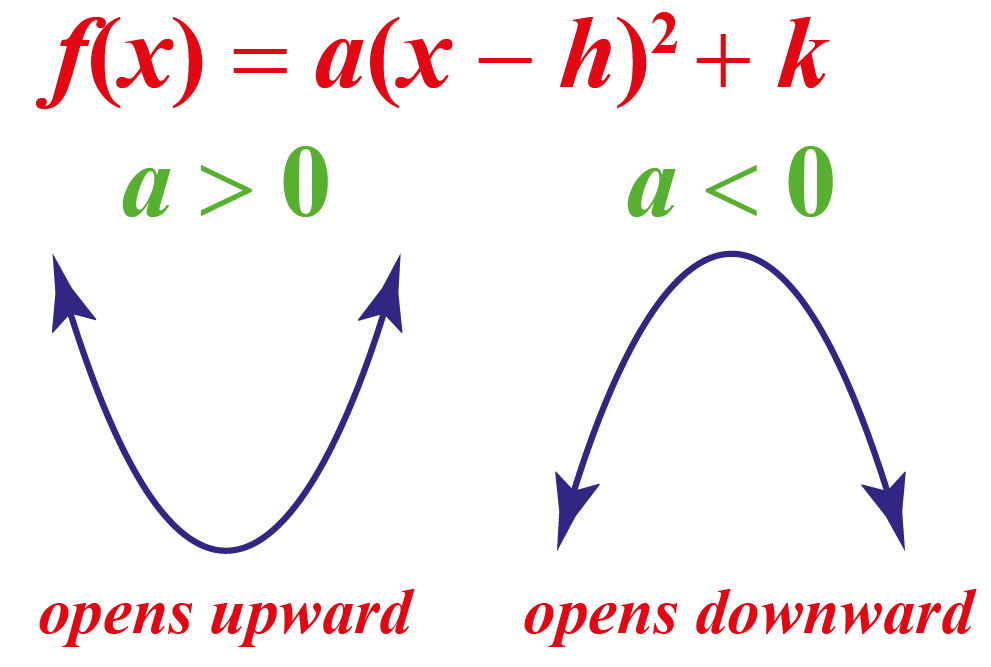### Quadratic Functions and Equations

An equation of the form $$ax^2+bx+c=0$$, where $$a \neq 0$$ is called a quadratic equation.

So, by a quadratic equation, we mean all the values of $$x$$ for which the quadratic function is zero.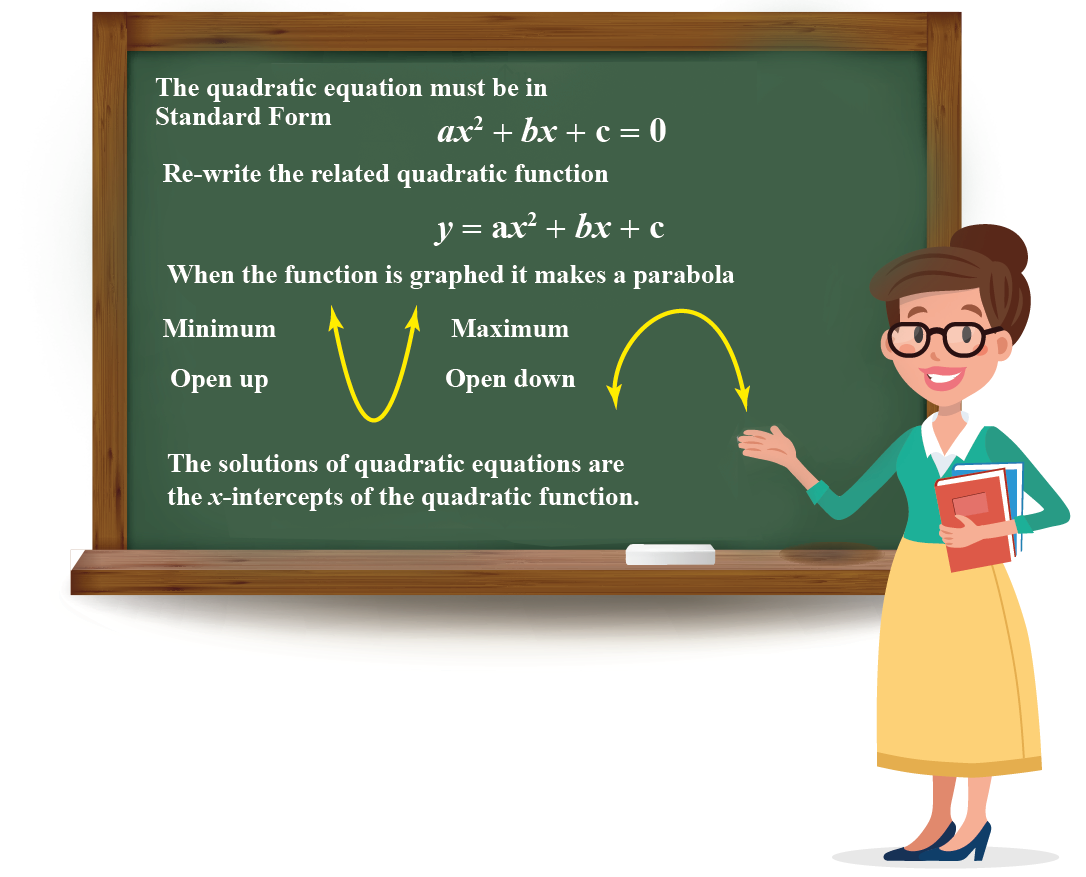## What Is the Quadratic Formula?

Sometimes working with a hard quadratic function is difficult. But don’t worry! Quadranator is here to help.

Quadranator alone is enough to hunt down all the solutions of the quadratic functions.

The quadratic functions formula is: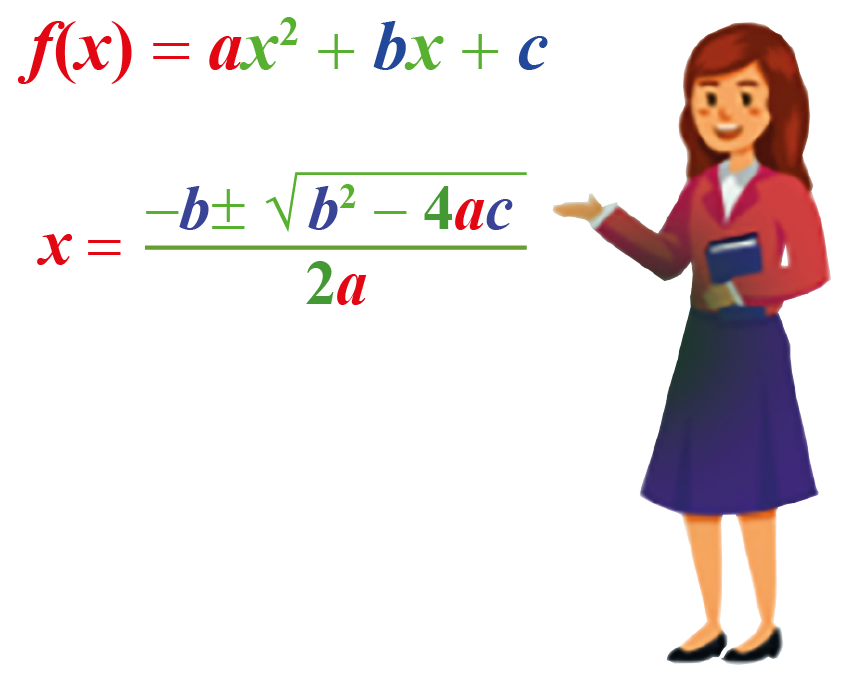This is also called a quadratic formula.

Do you have any difficulty memorizing the quadratic formula?

Don't worry! A rhyme or a song is the best way to remember it.

So, just sing along to the tune of “Pop Goes the Weasel.”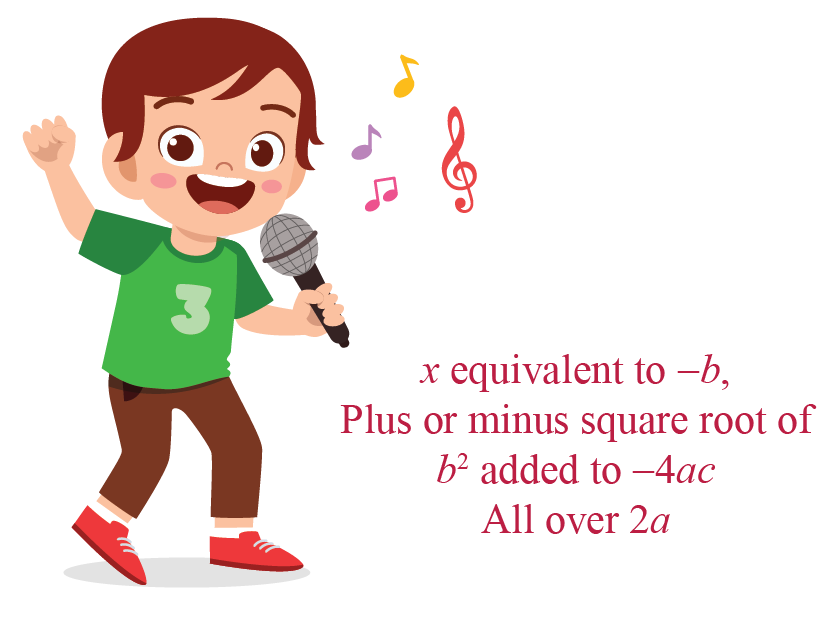Download Functions Worksheets
Functions
grade 10 | Answers Set 1
Functions
grade 10 | Answers Set 2
Functions
grade 10 | Questions Set 1
Functions
grade 10 | Questions Set 2
More Important Topics
Numbers
Algebra
Geometry
Measurement
Money
Data
Trigonometry
Calculus